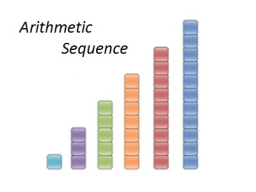# The sum 23

The sum of the first 10 terms of an arithmetic sequence is 530. What is the first term if the last term is 80? What is the common difference?

a1 =  26
d =  6

### Step-by-step explanation:Did you find an error or inaccuracy? Feel free to write us. Thank you!